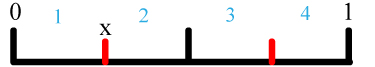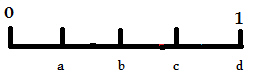Name:    3rd Grade Number & Operations—FractionsDevelop understanding of fractions as numbers.  Standard 3abc, Test 1

Multiple Choice
Identify the choice that best completes the statement or answers the question.

1.

What is the equivalent (equal) fraction of 1/3?
 a. 1/6 c. 2/6 b. 3/1 d. 1/2

2.

What is the equivalent (equal) fraction of 4/5?
 a. 2/3 c. 4/1 b. 8/10 d. 1

3.

What is the equivalent (equal) fraction of 2/4?
 a. 1/2 c. 2/8 b. 4/5 d. 1

4.

Which of the line diagrams below is equivalent to 3/4
 a.c.b.d. None are equivalent

Completion
Complete each statement.

5.

Write the next higher equivalent fraction for  2/5

6.

Write the next higher equivalent fraction for  2/3

7.

Write the next lower equivalent fraction for  3/6

8.

Write the next lower equivalent fraction for  5/10

9.

What is the equivalent whole number for 6/1?

10.

Write the simplest (smallest numbers possible) fraction equivalent to 3.

11.Which letter on the number line above equals 4/4?